Optimal Allocation

When you specify the ALLOC=OPTIMAL option in the STRATA statement, PROC SURVEYSELECT allocates the total sample size among the strata in proportion to stratum sizes, stratum costs, and stratum variances. You provide the stratum costs and variances by using the COST= and VAR= options, respectively.

Optimal allocation minimizes the overall variance for a specified cost, or equivalently minimizes the overall cost for a specified variance. See Lohr (2010), Cochran (1977), and Kish (1965) for details. For optimal allocation, PROC SURVEYSELECT computes the proportion of the total sample size for stratumas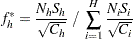where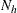is the number of sampling units in stratum,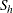is the standard deviation within stratum,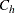is the unit cost within stratum, and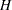is the total number of strata.

If you specify the total sample size n in the SAMPSIZE= option in the PROC SURVEYSELECT statement, the procedure computes the target sample size for stratumas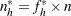As described in the section Proportional Allocation, the values of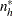are converted to integer sample sizes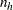by using a rounding algorithm that requires the sum of the stratum sample sizes to equal. The final stratum sample sizesare also required to be at least 1, or at least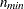if you specify a minimum stratum sample size in the ALLOCMIN= option in the STRATA statement. For without-replacement selection methods, the final sample sizes cannot exceed the stratum sizes.